Create a line plot.

ggline(
data,
x,
y,
group = 1,
numeric.x.axis = FALSE,
combine = FALSE,
merge = FALSE,
color = "black",
palette = NULL,
linetype = "solid",
plot_type = c("b", "l", "p"),
size = 0.5,
shape = 19,
stroke = NULL,
point.size = size,
point.color = color,
title = NULL,
xlab = NULL,
ylab = NULL,
facet.by = NULL,
panel.labs = NULL,
short.panel.labs = TRUE,
select = NULL,
remove = NULL,
order = NULL,
error.plot = "errorbar",
label = NULL,
font.label = list(size = 11, color = "black"),
label.select = NULL,
repel = FALSE,
label.rectangle = FALSE,
show.line.label = FALSE,
position = "identity",
ggtheme = theme_pubr(),
...
)

## Arguments

data

a data frame

x, y

x and y variables for drawing.

group

grouping variable to connect points by line. Allowed values are 1 (for one line, one group) or a character vector specifying the name of the grouping variable (case of multiple lines).

numeric.x.axis

logical. If TRUE, x axis will be treated as numeric. Default is FALSE.

combine

logical value. Default is FALSE. Used only when y is a vector containing multiple variables to plot. If TRUE, create a multi-panel plot by combining the plot of y variables.

merge

logical or character value. Default is FALSE. Used only when y is a vector containing multiple variables to plot. If TRUE, merge multiple y variables in the same plotting area. Allowed values include also "asis" (TRUE) and "flip". If merge = "flip", then y variables are used as x tick labels and the x variable is used as grouping variable.

color

line colors.

palette

the color palette to be used for coloring or filling by groups. Allowed values include "grey" for grey color palettes; brewer palettes e.g. "RdBu", "Blues", ...; or custom color palette e.g. c("blue", "red"); and scientific journal palettes from ggsci R package, e.g.: "npg", "aaas", "lancet", "jco", "ucscgb", "uchicago", "simpsons" and "rickandmorty".

linetype

line type.

plot_type

plot type. Allowed values are one of "b" for both line and point; "l" for line only; and "p" for point only. Default is "b".

size

Numeric value (e.g.: size = 1). change the size of points and outlines.

shape

point shapes.

stroke

point stroke. Used only for shapes 21-24 to control the thickness of points border.

point.size

point size.

point.color

point color.

title

plot main title.

xlab

character vector specifying x axis labels. Use xlab = FALSE to hide xlab.

ylab

character vector specifying y axis labels. Use ylab = FALSE to hide ylab.

facet.by

character vector, of length 1 or 2, specifying grouping variables for faceting the plot into multiple panels. Should be in the data.

panel.labs

a list of one or two character vectors to modify facet panel labels. For example, panel.labs = list(sex = c("Male", "Female")) specifies the labels for the "sex" variable. For two grouping variables, you can use for example panel.labs = list(sex = c("Male", "Female"), rx = c("Obs", "Lev", "Lev2") ).

short.panel.labs

logical value. Default is TRUE. If TRUE, create short labels for panels by omitting variable names; in other words panels will be labelled only by variable grouping levels.

select

character vector specifying which items to display.

remove

character vector specifying which items to remove from the plot.

order

character vector specifying the order of items.

character vector for adding another plot element (e.g.: dot plot or error bars). Allowed values are one or the combination of: "none", "dotplot", "jitter", "boxplot", "point", "mean", "mean_se", "mean_sd", "mean_ci", "mean_range", "median", "median_iqr", "median_hilow", "median_q1q3", "median_mad", "median_range"; see ?desc_statby for more details.

parameters (color, shape, size, fill, linetype) for the argument 'add'; e.g.: add.params = list(color = "red").

error.plot

plot type used to visualize error. Allowed values are one of c("pointrange", "linerange", "crossbar", "errorbar", "upper_errorbar", "lower_errorbar", "upper_pointrange", "lower_pointrange", "upper_linerange", "lower_linerange"). Default value is "pointrange" or "errorbar". Used only when add != "none" and add contains one "mean_*" or "med_*" where "*" = sd, se, ....

label

the name of the column containing point labels. Can be also a character vector with length = nrow(data).

font.label

a list which can contain the combination of the following elements: the size (e.g.: 14), the style (e.g.: "plain", "bold", "italic", "bold.italic") and the color (e.g.: "red") of labels. For example font.label = list(size = 14, face = "bold", color ="red"). To specify only the size and the style, use font.label = list(size = 14, face = "plain").

label.select

can be of two formats:

• a character vector specifying some labels to show.

• a list containing one or the combination of the following components:

• top.up and top.down: to display the labels of the top up/down points. For example, label.select = list(top.up = 10, top.down = 4).

• criteria: to filter, for example, by x and y variabes values, use this: label.select = list(criteria = "y > 2 & y < 5 & x %in% c('A', 'B')").

repel

a logical value, whether to use ggrepel to avoid overplotting text labels or not.

label.rectangle

logical value. If TRUE, add rectangle underneath the text, making it easier to read.

show.line.label

logical value. If TRUE, shows line labels.

position

Position adjustment, either as a string naming the adjustment (e.g. "jitter" to use position_jitter), or the result of a call to a position adjustment function. Use the latter if you need to change the settings of the adjustment.

ggtheme

function, ggplot2 theme name. Default value is theme_pubr(). Allowed values include ggplot2 official themes: theme_gray(), theme_bw(), theme_minimal(), theme_classic(), theme_void(), ....

...

other arguments to be passed to geom_dotplot.

## Details

The plot can be easily customized using the function ggpar(). Read ?ggpar for changing:

• main title and axis labels: main, xlab, ylab

• axis limits: xlim, ylim (e.g.: ylim = c(0, 30))

• axis scales: xscale, yscale (e.g.: yscale = "log2")

• color palettes: palette = "Dark2" or palette = c("gray", "blue", "red")

• legend title, labels and position: legend = "right"

• plot orientation : orientation = c("vertical", "horizontal", "reverse")

ggpar, ggbarplot

## Examples

# Data
df <- data.frame(dose=c("D0.5", "D1", "D2"),
len=c(4.2, 10, 29.5))
print(df)
#>   dose  len
#> 1 D0.5  4.2
#> 2   D1 10.0
#> 3   D2 29.5

# Basic plot
# +++++++++++++++++++++++++++
ggline(df, x = "dose", y = "len")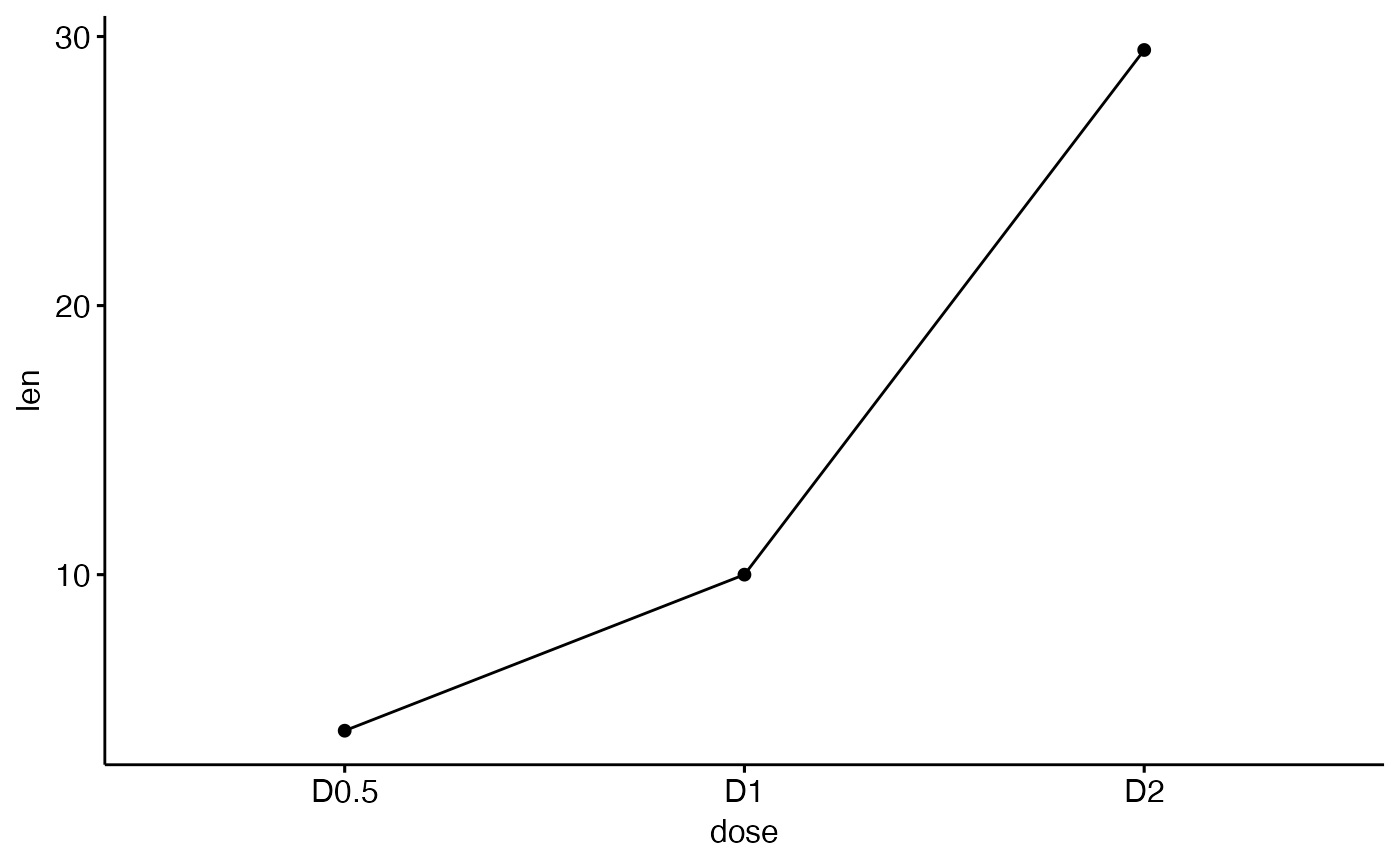# Plot with multiple groups
# +++++++++++++++++++++

# Create some data
df2 <- data.frame(supp=rep(c("VC", "OJ"), each=3),
dose=rep(c("D0.5", "D1", "D2"),2),
len=c(6.8, 15, 33, 4.2, 10, 29.5))
print(df2)
#>   supp dose  len
#> 1   VC D0.5  6.8
#> 2   VC   D1 15.0
#> 3   VC   D2 33.0
#> 4   OJ D0.5  4.2
#> 5   OJ   D1 10.0
#> 6   OJ   D2 29.5

# Plot "len" by "dose" and
# Change line types and point shapes by a second groups: "supp"
ggline(df2, "dose", "len",
linetype = "supp", shape = "supp")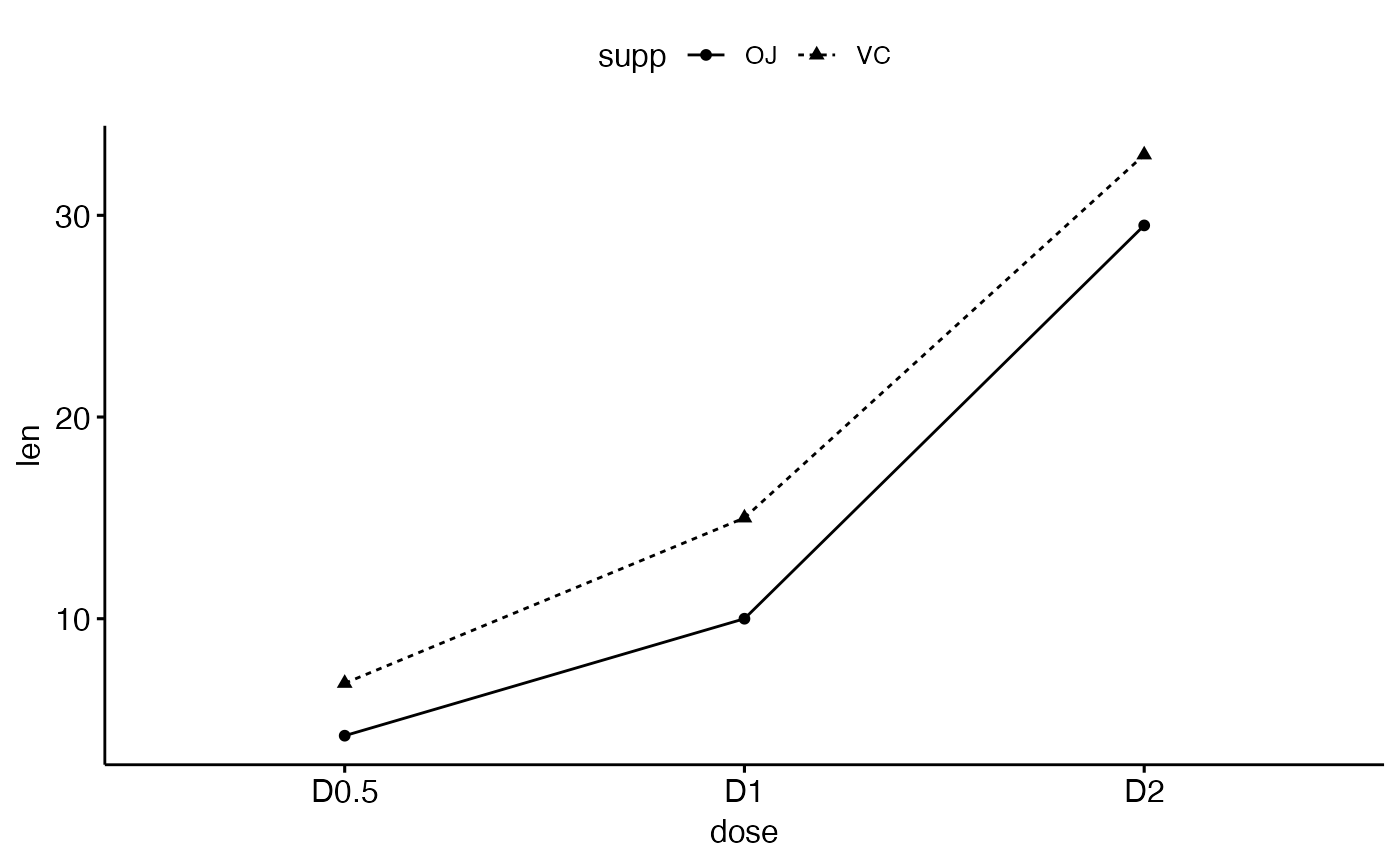# Change colors
# +++++++++++++++++++++

# Change color by group: "supp"
# Use custom color palette
ggline(df2, "dose", "len",
linetype = "supp", shape = "supp",
color = "supp", palette = c("#00AFBB", "#E7B800"))# ++++++++++++++++++++++++++

# Data: ToothGrowth data set we'll be used.
df3 <- ToothGrowth
#>     len supp dose
#> 1   4.2   VC  0.5
#> 2  11.5   VC  0.5
#> 3   7.3   VC  0.5
#> 4   5.8   VC  0.5
#> 5   6.4   VC  0.5
#> 6  10.0   VC  0.5
#> 7  11.2   VC  0.5
#> 8  11.2   VC  0.5
#> 9   5.2   VC  0.5
#> 10  7.0   VC  0.5

# It can be seen that for each group we have
# different values
ggline(df3, x = "dose", y = "len")# Visualize the mean of each group
ggline(df3, x = "dose", y = "len",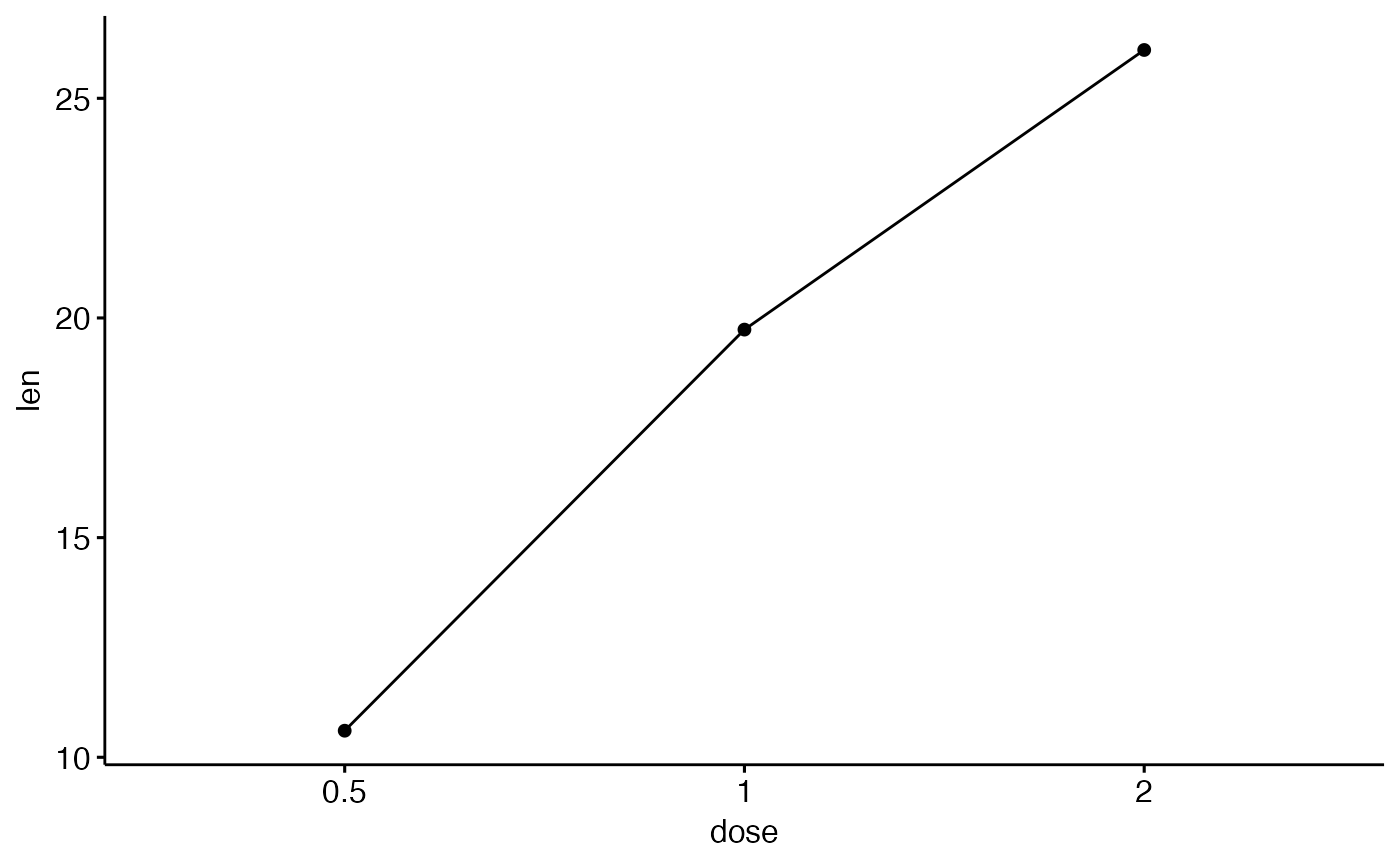# (other values include: mean_sd, mean_ci, median_iqr, ....)
ggline(df3, x = "dose", y = "len", add = "mean_se")# Change error.plot to "pointrange"
ggline(df3, x = "dose", y = "len",
add = "mean_se", error.plot = "pointrange")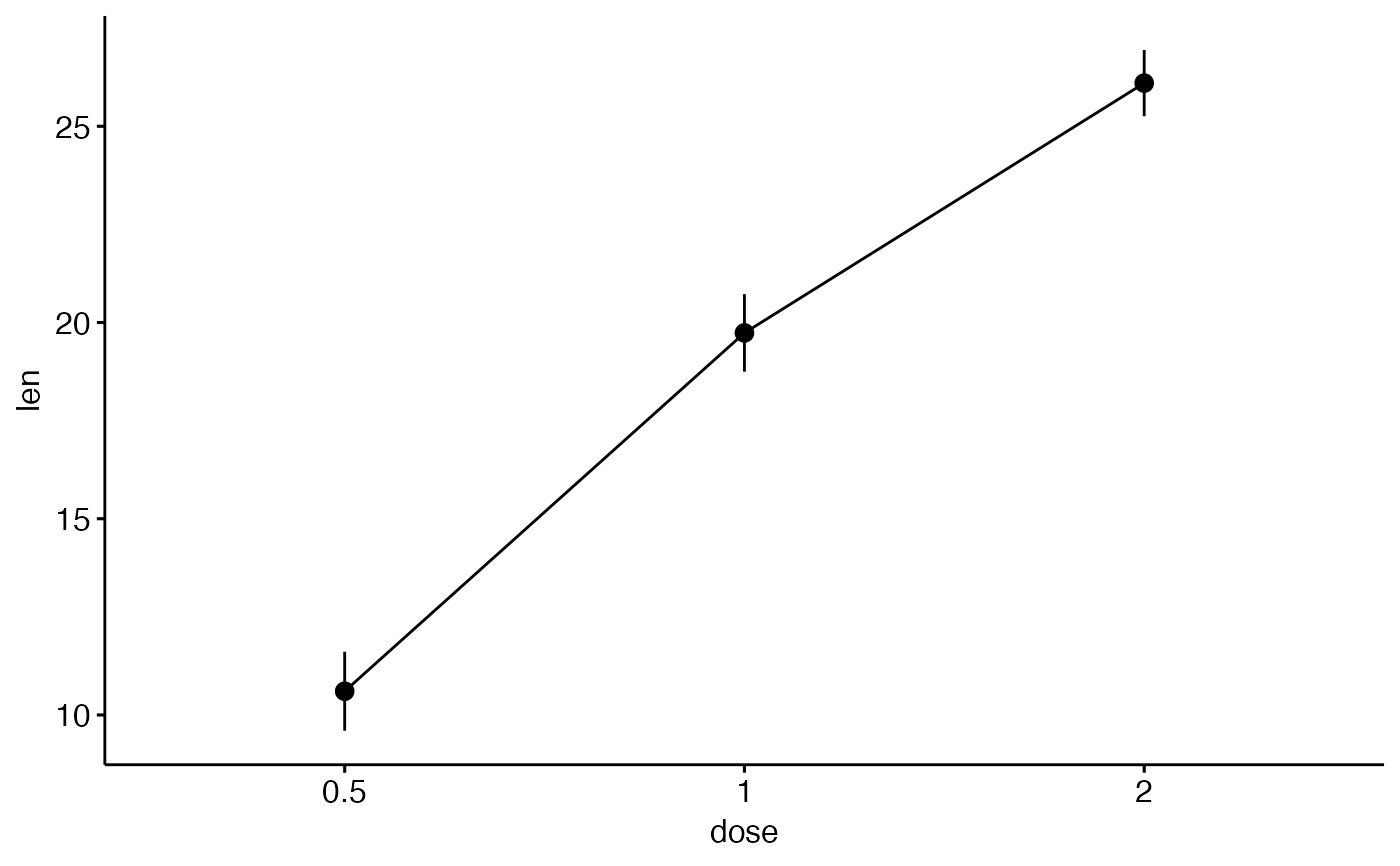# Add jitter points and errors (mean_se)
ggline(df3, x = "dose", y = "len",# Add dot and errors (mean_se)
ggline(df3, x = "dose", y = "len",
add = c("mean_se", "dotplot"), color = "steelblue")
#> Bin width defaults to 1/30 of the range of the data. Pick better value with
#> binwidth.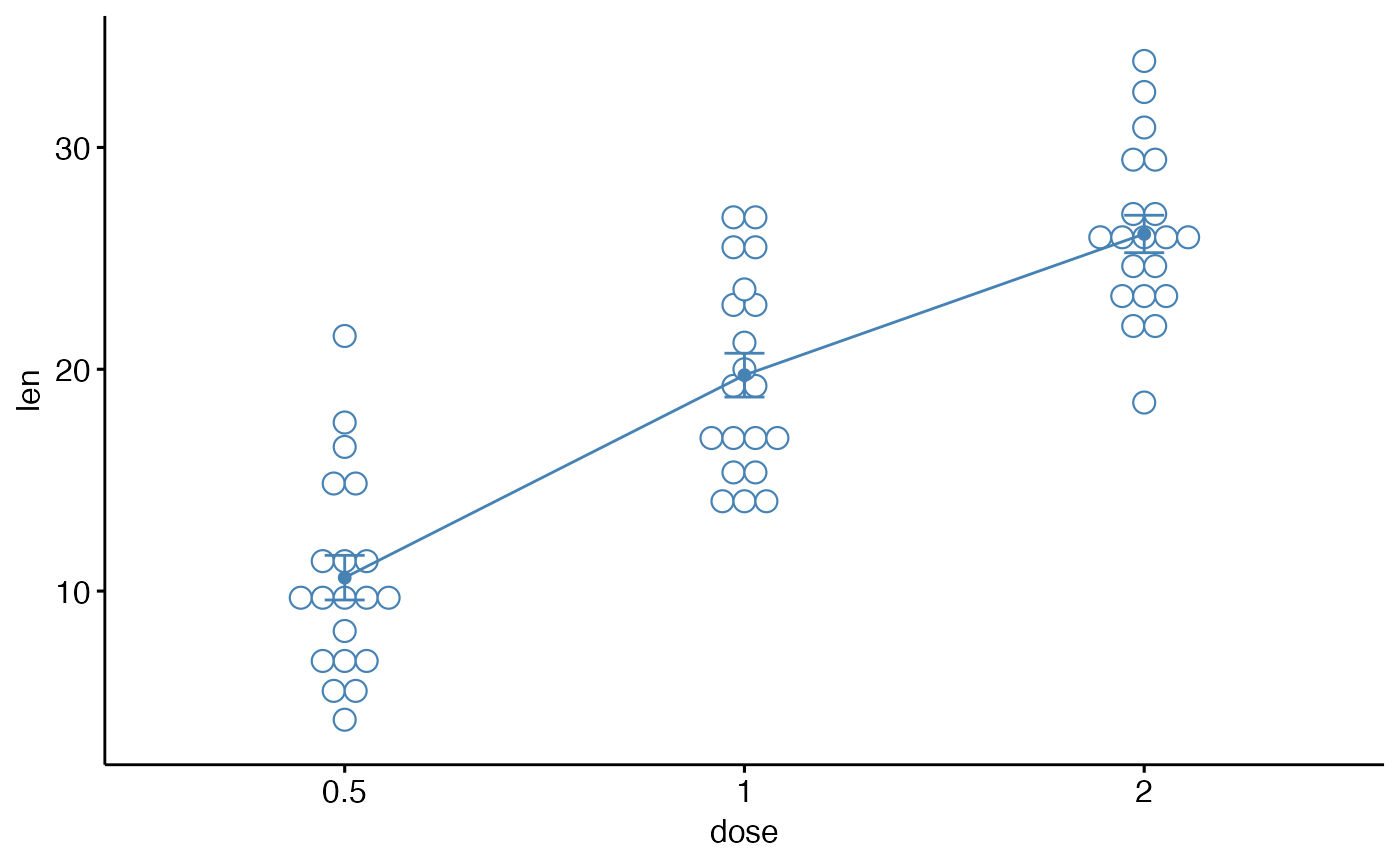# Add violin and errors (mean_se)
ggline(df3, x = "dose", y = "len",
add = c("mean_se", "violin"), color = "steelblue")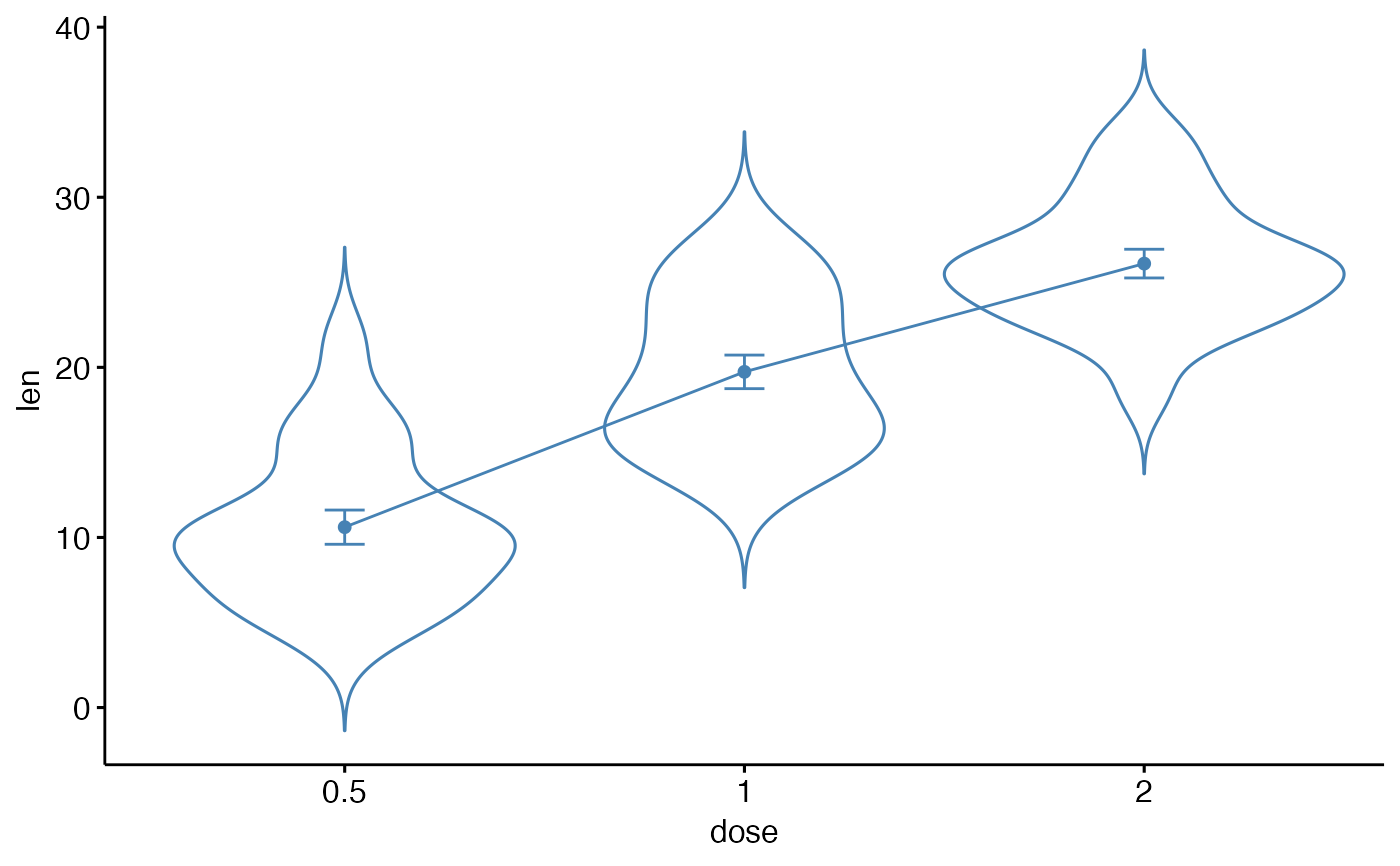# Multiple groups with error bars
# ++++++++++++++++++++++

ggline(df3, x = "dose", y = "len", color = "supp",
add = "mean_se", palette = c("#00AFBB", "#E7B800"))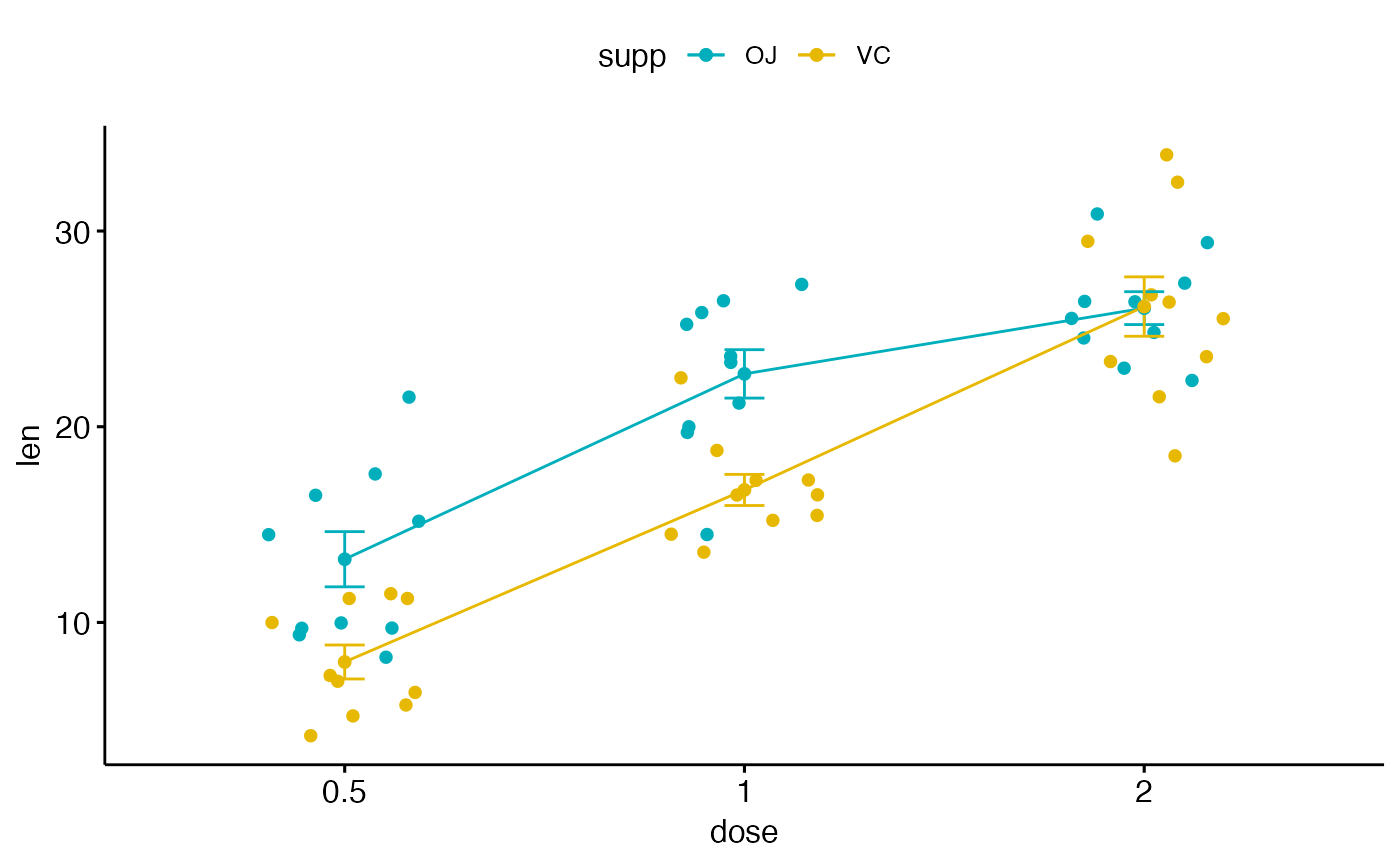#> binwidth.